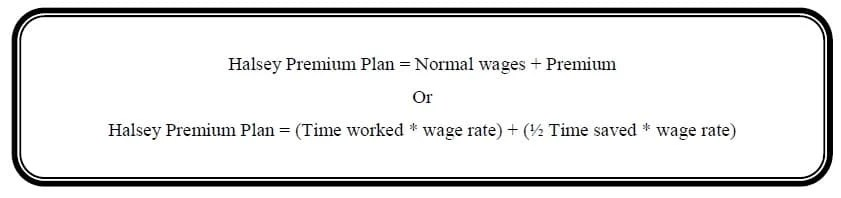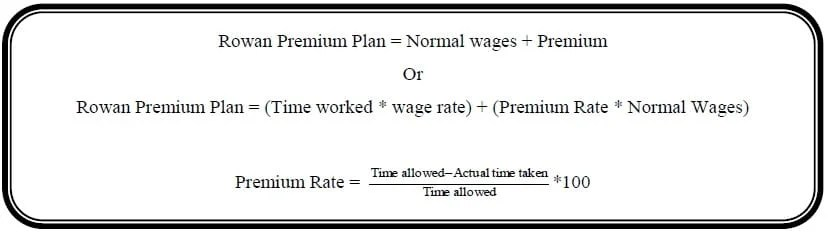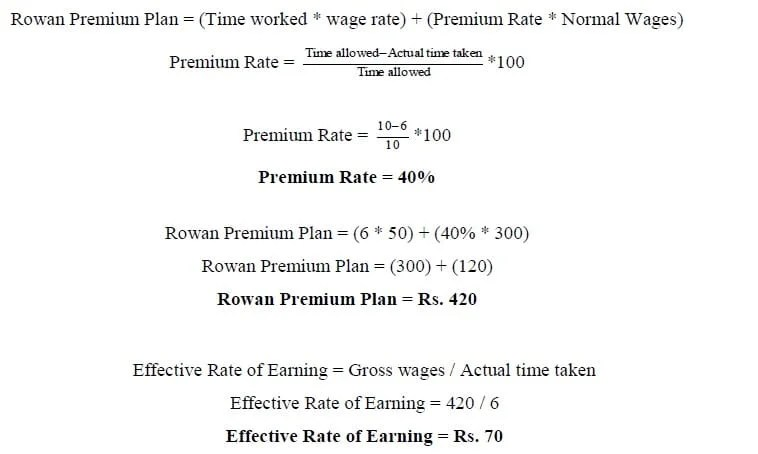Select Page

# Payroll Systems

Previous Lesson: Labor Cost

Different payroll systems have been devised for meeting the requirements of both employees and employers. Usually the employer makes a specified monetary payment to workers for a specified work or job done. The specified monetary payment could either be related to a particular hours of attendance or to the quantity of work.

• Time Rate Based Premium Plan
• Piece Rate Based Premium Plan

Under this system, wages to a worker are paid on time basis irrespective of the quantity of production. The wage is measured on the basis of unit of time i.e. hourly, daily, weekly or monthly. Some of the important time based incentive wage plans are briefly discussed below with practical examples:

Under this system, a standard time is fixed for each job or operation. Time rate is guaranteed to a worker, if he completes the job within standard time or more than the standard time, he is paid standard rate. But if the Job is completed in less than the standard time fixed for the job, he is given wages for the actual hours taken plus bonus equal to one half of the wage of the time saved:## Example 1:

Following are data related to payroll are extracted from the books of Ali Khan Ltd:

Wage rate per hour                   Rs. 50

Time allowed for the job           10 hours

Time taken                                  6 hours

Required: (a) Calculate the Gross wages using Halsey premium plan

(b) Find out Effective Rate of Earnings

### Solution:

Halsey Premium Plan = (Time worked * wage rate) + (½ Time saved * wage rate)

Halsey Premium Plan = (6 * 50) + (½ 4 * 50)

Halsey Premium Plan = (300) + (100)

Halsey Premium Plan = Rs. 400

Effective Rate of Earning = Gross wages / Actual time taken

Effective Rate of Earning = 400 / 6

Effective Rate of Earning = Rs. 66.67

>> Practice Multiple-choice Question Labor Costing MCQs.

## Video Lecture: Costing Concepts in Urdu & Hindi-Workbook Practice

Under this method, other things being the same as Halsey Plan, the rate of premium usually applied is 1/3 of the wage of the time saved:## Example 2:

Following are data related to payroll are extracted from the books of Ali Khan Ltd:

Wage rate per hour              Rs. 50

Time allowed for the job     10 hours

Time taken                           6 hours

Required: (a) Calculate the Gross wages using Halsey-Weir Premium Plan            (b) Find out Effective Rate of Earnings

### Solution:

Halsey-Weir Premium Plan = (Time worked * wage rate) + (1/3 Time saved * wage rate)

Halsey-Weir Premium Plan = (6 * 50) + (1/3 4 * 50)

Halsey-Weir Premium Plan = (300) + (66.67)

Halsey-Weir Premium Plan = Rs. 366.67

Effective Rate of Earning = Gross wages / Actual time taken

Effective Rate of Earning = 366.67 / 6

Effective Rate of Earning = Rs. 61.11

>> Practice Multiple-choice Question Labor Costing MCQs.

This system is similar to the above two plans. The worker is guaranteed at ordinary rate of wages and premium or bonus is paid in respect of time saved. Under the Rowan system the premium rate is calculated as the proportion of time saved to the standard time allowed.In order to get premium amount premium rate is multiplied by normal wages.## Example 3:

Following are data related to payroll are extracted from the books of Ali Khan Ltd:

Wage rate per hour              Rs. 50

Time allowed for the job      10 hours

Time taken                           6 hours

Required: (a) Calculate the Gross wages using Rowan Premium Plan     (b) Find out Effective Rate of Earnings

### Solution:## Example 4:

Following are data related to payroll are extracted from the books of Ali Ahmed Khan Ltd:

Wage rate per hour                  Rs. 10

Time allowed for the job          40 hours

Time taken                               30 hours

Required:

(a) Calculate the Gross wages using Halsey, Halsey-weir and Roman Premium plan

(b) Find out Effective Rate of Earnings by above mentioned three bonus plans and comparative wage sheet

Halsey Premium Plan = (Time worked * wage rate) + (½ Time saved * wage rate)

Halsey Premium Plan = (30 * 10) + (½ 10 * 10)

Halsey Premium Plan = (300) + (50)

Halsey Premium Plan = Rs. 350

Effective Rate of Earning = Gross wages / Actual time taken

Effective Rate of Earning = 350 / 30

Effective Rate of Earning = Rs. 11.67

Halsey-Weir Premium Plan = (Time worked * wage rate) + (1/3 Time saved * wage rate)

Halsey-Weir Premium Plan = (30 * 10) + (1/3 10 * 10)

Halsey-Weir Premium Plan = (300) + (33.33)

Halsey-Weir Premium Plan = Rs. 333.33

Effective Rate of Earning = Gross wages / Actual time taken

Effective Rate of Earning = 333.33 / 30

Effective Rate of Earning = Rs. 11.11>> Other Practice contains Cost of Goods Sold Problems and Solutions.

## Piece Rate Based Premium Plan

Under this method of remuneration a worker is paid on the basis of production and not time taken by him to perform the work. Piecework is where a fixed amount is paid per unit of output achieved irrespective of the time spend.This is one of the simplest and most commonly used of all incentive schemes. The rate is expressed in terms of certain sum of money for every unit produced, e.g. Rs 2 etc.

## Straight Piece Rate

• Wages are paid in this system in accordance with the output of production. This is independent of time spent on the job.

#### Straight Piece Rate Formula## Example 5:

If a worker produces 25 pieces per day and he is paid at the rate of Rs. 20 per piece, what will be the total wages per day?

### Solution:

Straight Piece Rate = Unit Produced * Rate Per Unit

Straight Piece Rate = 25 * 20

Straight Piece Rate = Rs. 500

## Piece-rate with guarantee

• A piece rate with guarantee operates to give the employee some security if the employee does not provide enough work in particular period
• If an employee’s earning for the amount of units produced in the periods are lower than guaranteed amount, then the guaranteed amount is paid

## Example 6:

Mr. Mohsin Khan is paid Rs. 3.00 for every unit that he produces but he has a guaranteed wage of Rs. 28.00 per eight-hour a day. In a particular week he produces the following number of units:

Monday …………………………     12 units                                  Tuesday …………………………     14 units

Wednesday ………………………    9 unit                                      Thursday ………………………..     18 units

Friday ……………………………     8 units

Calculate Mohsin’s wages of this week?

### Solution:## Differential piece rates

• There are various differential piece rate plans. The differential piece rate plans aim at maximum production by giving an additional incentive to increase output just at the stage at which the worker would otherwise begin to feel that further efforts was not worthwhile
• These schemes are devised in such a manner that with the increase in efficiency of a worker his wages are automatically increased. Some of the differential schemes are:
• Taylor Differential Piece Rate Plan
• Merrick Differential Piece Rate Plan

## Taylor differential piece rate system

• This system was originated by W.F. Taylor, the father of scientific management
• It is based on the assumption that the degree of efficiency varies from worker to worker and hence the worker must be paid according to their degree of efficiency
• Under this system two piece rates are fixed
• One lower rate applicable to worker whose production is below standard and another higher rate is applicable to a worker whose production is at or above the standard

## Example 7:

From the following particulars, calculate the earnings of workers under Taylor’s Differential Piece Rate Plan.

Standard production per day                                            27 pieces

Normal rate per piece                                                         80

A produces                                                                            25 units

B produces                                                                            35 units

Differential to be applied 80% of piece rate below standard. 120% of piece rate at or above standard

### Solution:

A

Per unit Rate = 80 * 80/100 = Rs. 64

Gross wages = 25 * 64 = Rs. 1,600

B

Per unit Rate = 80 * 120/100= Rs. 96

Gross wages = 96 * 35 = Rs. 3,360

## Merrick Differential Piece Rate System

• Merrick Differential system is a modification of the Taylor’s system
• Uses three rates instead of two. The three rates are as under:
• Efficiency upto 80% ………. Normal Piece rate applicable
• Efficiency upto 100% ……… 10% above normal rate
• Efficiency above 100% …….. 20% above normal rate

### Example 8:

From the following particulars calculate the total earnings of the three workers who are paid wages under the Merrick Differential System.

Normal piece rate (upto 80%)                                                           Rs. 5 per unit

Standard Production                                                                           40 units per week

Output of the workers for the week:

A                                             32 units

B                                             37 units

C                                             42 units

### Solution:

A

Efficiency Level = 32/40 * 100 = 80%

Gross wages = 32 * 5 = Rs. 160

B

Efficiency Level = 37/40 * 100 = 92.5%

Per unit Rate = 5 * 110/100 = Rs. 5.5

Gross wages = 37 * 5.5 = Rs. 203.5

C

Efficiency Level = 42/40 * 100 = 105%

Per unit Rate = 5 * 120/100 = Rs. 6.0

Gross wages = 42 * 6.0 = Rs. 252

>> Practice Multiple-choice Question Labor Costing MCQs.

## Labor Turnover

• Labour turnover may be defined as the rate of change in the composition of the labor force of an organization
• High rate of labor turnover denotes that labor is not stable and there is frequent change in the labor force
• The high labour turnover rate is an important indication of high labor cost. It is therefore not desirable

#### Labor Turnover Formula## Example 9:

The personnel department of a company has supplied the following information relating to its work force during the month of June, 2011.

Number of workers:

1st June …………….. 1900                                                                30th June …………… 2100

During the month 60 persons were discharged and 20 left the company. During the month 200workers were engaged out of which only 40 workers were appointed against the vacancy caused by the number of workers separated and the remaining on account of an expansion scheme of the company. Calculate labor turnover rate?

### Solution:## Labor Efficiency

• Labor is a significant cost in many organizations and it is important to measure the efficiency of labor against pre-set targets
• To measure labor efficiency compare actual efficiency with predetermined targets (or budgets)

#### Labor Efficiency Formula## Example 10:

ABC Company budgets to make 27,000 standard units of output (in four hour each) during a budget period of 108,000 hours. Actual output the period was 27,000 units which took 120,000 hours to make.  Find the Efficiency Ratio?

### Solution:>> Practice Multiple-choice Question Labor Costing MCQs.

### Related Topics

Inventory Management MCQs

Inventory Management Problems

Economic Order Quantity

Economic Order Quantity MCQ

Economic Order Quantity Problems

### More Interest

Labor Cost

Inventory Management

Labor Cost MCQs

Labor Cost Problems

Inventory Valuation

## References

Ramchandran, N., & Kakani, R. K. (2007). Financial Accounting for Management. (2nd, Ed.) New Delhi: Tata McGraw Hill.

Sehgal, A., & Sehgal, D. (n.d.). Advanced Accountancy (Vol. I & II). New Delhi: Taxmann Publication Pvt. Ltd.

Shukla, M. C., Grewal, T. S., & Gupta, S. C. (2008). Advanced Accountancy (Vol. I & II). New Delhi: S Chand & Co.

Weygandt, J. J., Kimmel, P. D., & Kieso, D. E. (2012). Accounting Principles (10th ed.). Hoboken: John Wiley & Sons, Inc.

Williams, M., & Bettner, H. (1999). Accounting (The basic for business decisions). (11th, Ed.) USA: Irwin McGraw- Hill.